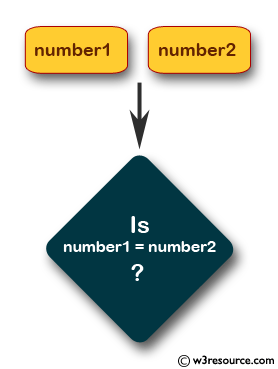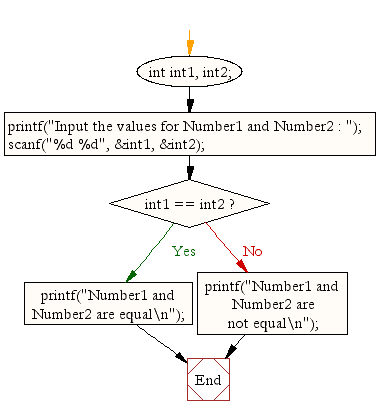﻿ C Program: Check whether two integers are equal or not - w3resource# C Exercises: Check whether two integers are equal or not

## C Conditional Statement: Exercise-1 with Solution

Write a C program to accept two integers and check whether they are equal or not.

Pictorial Presentation:Sample Solution:

C Code:

``````#include <stdio.h>
void main()
{
int int1, int2;

printf("Input the values for Number1 and Number2 : ");
scanf("%d %d", &int1, &int2);
if (int1 == int2)
printf("Number1 and Number2 are equal\n");
else
printf("Number1 and Number2 are not equal\n");
}
```
```

Sample Output:

```Input the values for Number1 and Number2 : 15 15
Number1 and Number2 are equal
```

Flowchart:C Programming Code Editor:

Improve this sample solution and post your code through Disqus.

What is the difficulty level of this exercise?

Test your Programming skills with w3resource's quiz.

﻿

## C Programming: Tips of the Day

Why do C and C++ compilers allow array lengths in function signatures when they're never enforced?

It is a quirk of the syntax for passing arrays to functions.

Actually it is not possible to pass an array in C. If you write syntax that looks like it should pass the array, what actually happens is that a pointer to the first element of the array is passed instead.

Since the pointer does not include any length information, the contents of your [] in the function formal parameter list are actually ignored.

Ref : https://bit.ly/3fhlvdH